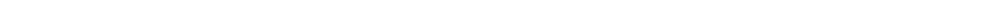Documents

# Tutorials

The role of the tutorials is to provide a platform for a more intensive scientific exchange amongst researchers interested in a particular topic and as a meeting point for the community. Tutorials complement the depth-oriented technical sessions by providing participants with broad overviews of emerging fields. A tutorial can be scheduled for 1.5 or 3 hours.

## TUTORIALS LIST

Tutorial on
A Functional Theory Framework for Neural Networks That Includes Training Data with an Introduction to TensorFlow 2.0 and Keras

InstructorUmberto Michelucci TOELT LLC, University of Portsmouth Switzerland Brief Bio Umberto studied physics and mathematics. He is an expert in numerical simulation, statistics, data science and machine learning. In addition to several years of research experience at the George Washington University (USA) and the University of Augsburg (DE), he has 15 years of practical experience in the fields of data warehouse, data science and machine learning. His last book “Applied Deep Learning – A Case-Based Approach to Understanding Deep Neural Networks” was published by Springer in 2018. He is currently working on his new book on “Convolutional and Recurrent Neural Networks Theory and Applications”. He is very active in research in the field of artificial intelligence. He publishes his research results regularly in leading journals and gives regular talks at international conferences. He teaches as lecturer at the ZHAW University of Applied Sciences for Deep Learning and Neural Networks Theory and Applications and at the HWZ University of economic science for Big Data Analysis and statistics. He is also responsible at Helsana Vesicherung AG for research and collaborations with universities in the area of AI. He just recently founded TOELT GmbH, a company aiming to develop new and modern teaching, coaching and research methods for AI, to make AI technologies and research accessible to every company and everyone.
Abstract

Abstract
In this tutorial I would like to present a complete review of the functional theory framework that helps us in understanding the problem if neural networks are universal approximators and why we can use as we do. Starting from Kolmogorov's Theorem and going thruogh results from Cybenko and Hanin (the last results are from 2017) I will explain the functional theory (and in particular in terms of metric spaces) of neural networks. In particular I will highlight the differences between the theory and how in practice are neural networks trained. In particular we will look at the role of the cost function (that plays the role of the metric) and at training methods. We will also show what metrics can be used for regression and a generalised metric for classification that reduces to the classical cross entropy when used for binary classification. We will also look at what part of this framework is influenced by the training dataset and why choosing different data will give different results (apart from the obvious reasons), since changing the training data will change the metric space in which we act. In addition I plan to give an overview of TensorFlow 2.0 and how to use it for research, giving tips and hints on usage of Keras. Exercises and a complete GitHub repository with jupyter notebooks will be provided. GitHub repository: https://github.com/toelt-llc/ICAART-Tutorial-Michelucci

Keywords
Machine Learning, Functional Analysis, neural networks, TensorFlow 2.0, Theory

Aims and Learning Objectives
The aim is to learn the fundamental theorems about neural networks that allows practitioners to use them as they do. We will learn why we can use neural networks for regression (or classification) and what hypothesis we implicitly do without mentioning them when we write programs that train neural networks. Additionally I will show a functional theory framework that will include neural network architecture and training dataset. Additionally the participants will learn the basics of TensorFlow 2.0 and Keras and how to apply it to their own projects.

Target Audience
Practitioners interested in the mathematical theory of neural networks. Mathematicians that study the problem of learning and computer scientist that wants to understand the fundamentals properties of neural networks.

Prerequisite Knowledge of Audience
Basic understanding of Analysis and mathematics at the undergraduate level. I will cover the basics of functional theory at the beginning. Basic understanding of how neural networks are working is an advantage. But I will also briefly cover that in the beginning. Basic to intermediate knowledge of Python is needed.

Detailed Outline
Neural network introduction (a more mathematical view) - Neural network introduction (a programming view with code snippets in Tensorflow and Python) - Functional theory introduction - Review of the theory from the 80s to today of the theory that describe the problem of under which conditions are network universal approximators. - Description of the framework for neural networks and some new metric that allows to discuss about regression and classification in terms of metric spaces - Introduction to Computational Graphs - Introduction to TensorFlow 2.0 - Introduction to Keras - practical examples and exercises in Python that the students can do live at the tutorial.

Secretariat Contacts
e-mail: icaart.secretariat@insticc.org